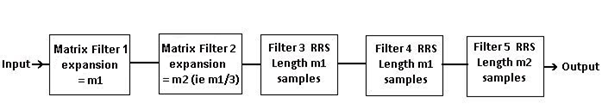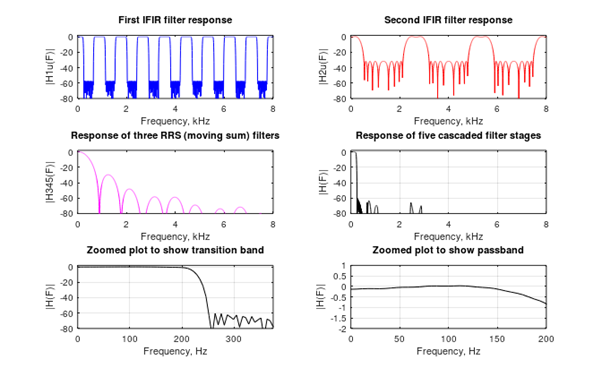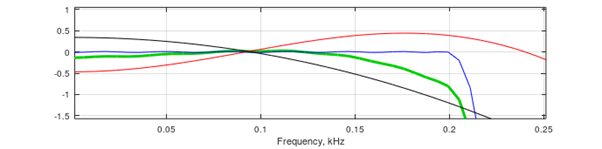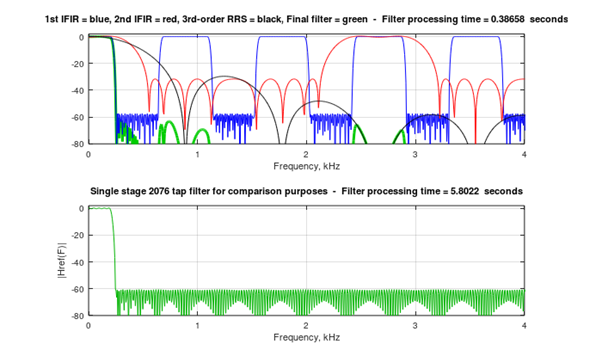# An Efficient Lowpass Filter in Octave

November 6, 2019

This article describes an efficient linear-phase lowpass FIR filter, coded using the Octave programming language. The intention is to focus on the implementation in software, but references are provided for those who wish to undertake further study of interpolated FIR filters - .

The input signal is processed as a vector of samples (eg from a .wav file), which are converted to a matrix format.   The complete filter is thus referred to as a Matrix IFIR or MIFIR.Fig 1. The filter has two band-edge shaping subfilters and three RRS (moving sum) stages.

For the example parameters provided (200 Hz cutoff frequency and 48 kHz sampling rate), the 5-stage design featured here was approximately 15 times faster than a single stage FIR filter. This test was run with Octave 5.1 on Windows 10 . Included in the code is a conventional single stage FIR filter, so that comparisons can be made in terms of processing time on signal blocks.

The code will also run on Matlab R2016b (possibly with some minor modifications for other versions). Although Matlab has an ‘IFIR’ function, this is not (at the time of writing) available in Octave, hence the alternative presented here.

At present, optimizing parameter values for a specific application is somewhat trial-and-error. That said, setting the required passband and stopband frequencies should provide a suitable starting point. Also, it is recommended to initially use the existing values of variables ‘taps’ and ‘taps2’. These can then be increased if a smaller transition band and/or higher stopband attenuation is required.

The filter configuration (Fig 1) is designed for use where the cutoff frequency is less than about 5% of the signal sampling rate (Fs).

Input and output sample rates are equal. Also, the data sample rate remains constant throughout the filter.

THEORY OF OPERATION

The filter (Fig 1) consists of two matrix band-edge shaping subfilters, followed by three recursive running sum (RRS) stages. For the parameters used in the example (200 Hz cutoff frequency and 48 kHz sample rate), this results in the plots of Fig 2.Fig 2.     Frequency response of filter stages for a 200 Hz passband and 48 kHz sample rate

Most implementations of IFIR filters have a single band-edge shaping stage, followed by further subfilter(s) to reduce the amplitude of image components. However this one has two band-edge shaping stages because :

(a)  The second stage, to some extent, masks the image components from the first stage. This masking is achieved most effectively when the expansion factors are related by :

m1 = 3 * m2

where m1 and m2 are the first and second stage expansion factors.

(b)  The second subfilter also has a slight upward amplitude characteristic in the passband frequency response, which partially counteracts droop in the first stage and RRS stages. This gives a flatter overall passband response, than would be the case for a single shaping stage.

Fig 3 shows the passband response for each stage of the filter, and the final result .

1st Matrix filter = blue, 2nd Matrix filter = red, 3rd-order RRS = black, Final filter = greenFig 3. Passband response of filter stages for a 200 Hz passband and 48 kHz sample rate.

A rather unusual configuration is employed for coding the first two subfilters, which filter the signal as a 2-dimensional matrix rather than a vector.

The matrix has one dimension matching the expansion factor (ie m1 for the first stage), and the second dimension equal to the signal block length (sz), divided by m1. Conversion from vector to matrix and vice-versa uses the Octave ‘reshape’ command.

Following the first stage, the signal block is converted to a different size matrix with one dimension as m2, and the other dimension equal to sz/m2.

Some zero padding may be necessary if the initial length of the signal block is not divisible by both m1 and m2 to obtain an integer. This is incorporated in the Octave code.

The final three subfilter stages are each RRS (also known as moving sum). Here there is good news and bad news. The good news is that a ‘movsum’ function is available in Octave which appears to work. However, the bad news is that it seems to be extremely slow compared to the ‘cumsum’ function used in the code below.

Since the RRS functions are moving sum rather than moving average, the coefficients for the two matrix stages (b1 and b2), are multiplied by a scaling factor to give a passband output level of approximately 0 dB (unity passband gain) for the complete filter.

EXAMPLE PARAMETERS

The code listing below features parameters for a lowpass filter with a passband ripple of less than 1 dB up to 200 Hz, and an attenuation of greater than 60 dB at frequencies exceeding 250 Hz.

In general, the number of taps specified for the first stage will determine the passband ripple and stopband attenuation. The main effect of increasing the number of taps in the second stage, is to increase the overall stopband attenuation.

Processing times are measured for both the MIFIR and a reference single-stage filter to enable a comparison to be made. See Fig 4 and the Octave command window.Fig 4.          Comparison of the frequency response for 5-stage and single stage filters.

CONCLUSION

The proposed efficient lowpass filter in Figure 1, known as a MIFIR filter, has been designed as a compromise between complexity and performance, as is so often the case in engineering.  Comments and suggestions for adapting and/or improving the code are most welcome.

```%              Five stage FIR lowpass filter - with chirp test
% ============================================================
%                      Paul Lovell November 2019
% This program runs in Octave 5.1 using the 'remez' function
% from the Octave-Forge 'signal' package.
%
% The taps in the first and second stages can be set to any
% number > 3, as required. Higher stopband attenuation, and/or
% a sharper transition band will require more taps.
%
% Example parameters below are for passband of 200 Hz at < 1 dB
% ripple, and stopband of 250 Hz and above with > 60 dB attenuation.
% *********************** Input Parameters **********************
% Set the lowpass filter input parameters as required
% Example values are shown.
%
clear all;
Fs = 48000;                     % Set sample rate in Hz
psb = 200;                      % Set passband maximum frequency Hz
stb = 250;                      % Set stopband minimum frequency Hz
taps = 57;                      % Set taps for first stage matrix filter
taps2 = 13;                     % Set taps for second stag matrix filter
% Set the chirp test signal parameters as required.
%
chf = int32(Fs/2); % maximum chirp frequency
chd = 30; % chirp duration in seconds
% ******************** Generate chirp signal block ****************
dt = 1/Fs;
t = (dt:dt:chd);
sig = chirp(t,0,chd,chf)';           % chirp test signal
% soundsc(s,Fs);                     % audio output if required
sz = Fs * chd;                       % size of input signal block
% *********** Single stage filter for comparison purposes ********
f = [0 0.0084 0.0105 1];
b_ref = remez(2075, f, [1 1 0 0], [1 40]); fp = Fs/1000;
[Href,Fref] = freqz(b_ref,1,4096,fp); % Determine frequency response
ref = tic;
ref_filter_out = filter(b_ref,1,sig);
timeref = num2str(toc(ref));
disp([char(10) 'Processing with single 2076 tap filter ' ...
timeref ' seconds' char(10)]);
% ******* Calculate filter passband and stopband parameters *******
m2 = round(Fs/(6 * (psb+stb)));  % expansion for filter 2
m1 = 3 * m2;                     % expansion for filter 1
pf = double(2 * psb * m1)/Fs;    % normalize passband frequency
sf = pf * stb/psb;               % normalize stopband frequency
f = [0 pf sf 1];                 % MIFIR-1 design parameters
b1 = remez(taps-1, f, [1 1 0 0], [1 1]);
f = [0 0.199 0.401 1];           % MIFIR-2 design parameters
b2 = remez(taps2-1, f, [1 1 0 0], [1 2]);
scale = 1.02/sqrt(m1^2*m2);      % scaling factor
b1 = b1*scale; b2 = b2*scale;    % scale coefficients
% ************* Five stage filter process starts here ************
tstart = tic;
sig2 = [sig(:); zeros(mod(-numel(sig),m1),1)];        % zero padding
sg1 = filter(b1,1,reshape(sig2,m1,[])')';             % 1st stage (MIFIR)
sg2 = filter(b2,1,reshape(sg1,m2,[])')';              % 2nd stage (MIFIR)
sg3 = reshape(sg2,[],1);
sg3 = cumsum(sg3-[zeros(m1,1); sg3(1:end-m1)]);       % 3rd stage (RRS)
sg3 = cumsum(sg3-[zeros(m1,1); sg3(1:end-m1)]);       % 4th stage (RRS)
sig_out = cumsum(sg3-[zeros(m2,1); sg3(1:end-m2)]);   % 5th stage (RRS)
timefilt = num2str(toc(tstart));
disp(['Processing with 5 stage filter ' timefilt ' seconds']);
% ***************** Display frequency vs amplitude **************
b1 = b1/scale; b2 = b2/scale;         % Rescale to display MIFIRs
b3 = ones(m1,1)*scale; b4 = b3;       % Rescale to display RRS
b5 = ones(m2,1);
[H1,F1] = freqz(b1,1,4096,fp);        % Spectrum of prototype filter
b1u = upsample(b1,m1);
[H1u,F1] = freqz(b1u,1,4096,fp);      % Spectrum of matrix filter 1
[H2,F2] = freqz(b2,1,4096,fp);        % Spectrum of prototype filter
b2u = upsample(b2,m2);
[H2u,F2] = freqz(b2u,1,4096,fp);      % Spectrum of matrix filter 2
[H3,F3] = freqz(b3,1,4096,fp);        % Spectrum of filter 3 (RRS)
[H4,F4] = freqz(b4,1,4096,fp);        % Spectrum of filter 4 (RRS)
[H5,F5] = freqz(b5,1,4096,fp);        % Spectrum of filter 5 (RRS)
H345 = H3.*H4.*H5;                 % Response of the cascaded RRS stages
H = H1u.*H2u.*H3.*H4.*H5;          % The overall filter frequency response
figure(1);
xscale = Fs/2000;
subplot(3,2,1); plot(F1,20*log10(abs(H1u)), '-b');
title('First IFIR filter response'); ylabel('|H1u(F)|');
axis([0,xscale/3,-80,2]); xlabel('Frequency, k Hz');
subplot(3,2,2); plot(F2,20*log10(abs(H2u)), '-r');
title('Second IFIR filter response'); ylabel('|H2u(F)|');
axis([0,xscale/3,-80,2]); xlabel('Frequency, k Hz');
subplot(3,2,3); plot(F2,20*log10(abs(H345)), '-m') ;
title('Response of three RRS (moving sum) filters'); ylabel('|H345(F)|');
axis([0,xscale/3,-80,2]); xlabel('Frequency, k Hz');
subplot(3,2,4); plot(F1,20*log10(abs(H)), '-k');
title('Response of five cascaded filter stages'); ylabel('|H(F)|');
axis([0,xscale/3,-80,2]); grid on; xlabel('Frequency, k Hz');
subplot(3,2,5); plot(F1*1000,20*log10(abs(H)), '-k'); ylabel('|H(F)|');
title('Zoomed plot to show transition band'); axis([0,stb*1.5,-80,2]);
grid on; xlabel('Frequency, Hz');
subplot(3,2,6); plot(F1*1000,20*log10(abs(H)), '-k'); ylabel('|H(F)|');
title('Zoomed plot to show passband'); axis([0,psb,-2,1]);
grid on; xlabel('Frequency, Hz');
figure(2);
subplot(2,1,1);
plot(F1,20*log10(abs(H)), 'color', [0 0.8 0], 'linewidth', 3); hold on
plot(F1,20*log10(abs(H1u)), '-b'); hold on
plot(F2,20*log10(abs(H2u)), '-r'); hold on
plot(F2,20*log10(abs(H345)), '-k'); hold off
axis([0,xscale/6,-80,2]); grid on; xlabel('Frequency, k Hz');
timfilt =(['1st IFIR = blue, 2nd IFIR = red, 3rd-order RRS = black,' ...
' Final filter = green' ...
' - Filter processing time = ' timefilt ' seconds']);
title(timfilt);
subplot(2,1,2);
plot(Fref/1.024,20*log10(abs(Href)), 'color',[0 0.7 0]);
ylabel('|Href(F)|');
timref = (['Single stage 2076 tap filter for comparison purposes', ...
' - Filter processing time = ' timeref ' seconds']);
title(timref);
axis([0,xscale/6,-80,2]);grid on; xlabel('Frequency, kHz');
% *********************************************************************
% This software is provided "AS IS", without warranty. The author shall not be liable for
% any claim, damages or other liability arising from, or in connection with this software.
```

REFERENCES AND ACKNOWLEDGEMENTS

My thanks to Richard (Rick) Lyons for assistance with Matlab testing and graphics implementation.

 Lyons, R., " Interpolated narrowband lowpass FIR filters",

IEEE Signal Processing Magazine, 20(1), January 2003, pp. 50-57. Available online at:

 Lyons, R., Streamlining Digital Signal Processing: A Tricks of the Trade Guidebook, 2nd Edition., Wiley-IEEE Press, 2012,Section 8.2.

 Vaidyanathan, P. Multirate Systems and Filter Banks, Prentice Hall, 1993, pp. 134-138.

To post reply to a comment, click on the 'reply' button attached to each comment. To post a new comment (not a reply to a comment) check out the 'Write a Comment' tab at the top of the comments.